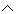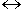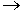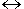# TautologiesExample 1: What do you notice about each sentence below?

 1 A number is even or a number is not even. 2 Cheryl passes math or Cheryl does not pass math. 3 It is raining or it is not raining. 4 A triangle is isosceles or a triangle is not isosceles.

Each sentence in Example 1 is the disjunction of a statement and its negation  Each of these sentences can be written in symbolic form as p~p. Recall that a disjunction is false if and only if both statements are false; otherwise it is true. By this definition, p~p is always true, even when statement p is false or statement ~p is false!  This is illustrated in the truth table below:

 p ~p p~p T F T F T T

The compound statement p~p consists of the individual statements p and ~p. In the truth table above, p~p is always true, regardless of the truth value of the individual statements. Therefore, we conclude that p~p is a tautology.

Definition: A compound statement, that is always true regardless of the truth value of the individual statements, is defined to be a tautology.

Let's look at another example of a tautology.

Example 2: Is (pq)p a tautology?

 p q pq (pq)p T T T T T F F T F T F T F F F T

Solution: The compound statement (pq)p consists of the individual statements p, q, and pq. The truth table above shows that (pq)p is true regardless of the truth value of the individual statements. Therefore, (pq)p is a tautology.

In the examples below, we will determine whether the given statement is a tautology by creating a truth table.

Example 3: Is x(xy) a tautology?

 x y xy x(xy) T T T T T F T T F T T T F F F T

Solution: Yes; the truth values of x(xy) are {T, T, T, T}.

Example 4: Is ~bb a tautology?

 b ~b ~bb T F T F T F

Solution: No; the truth values of ~bb are {T, F}.

Example 5: Is (pq)(pq) a tautology?

 p q (pq) (pq) (pq)(pq) T T T T T T F T F F F T T F F F F F F T

Solution: No; the truth values of (pq)(pq) are {T, F, F, T}.

Example 6: Is [(pq)p]p a tautology?

 p q pq (pq)p [(pq)p]p T T T T T T F F F T F T T F T F F T F T

Solution: Yes; the truth values of [(pq)p]p are {T, T, T, T}.

Example 7: Is (rs)(sr) a tautology?

 r s rs sr (rs)(sr) T T T T T T F F T F F T T F F F F T T T

Solution: No; the truth values of (rs)(sr) are {T, F, F, T}.Summary: A compound statement that is always true, regardless of the truth value of the individual statements, is defined to be a tautology. We can construct a truth table to determine if a compound statement is a tautology.

### Exercises

 1. What is the truth value of r~r? TrueFalseNot enough information was given.None of the above. RESULTS BOX:
 2. Is the following statement a tautology?  s~s YesNoNot enough information was given.None of the above. RESULTS BOX:
 3. Is the following statement a tautology? [(pq)~p]q YesNoNot enough information was given.None of the above. RESULTS BOX:
 4. Is the following statement a tautology? ~(xy)(~x~y) YesNoNot enough information was given.None of the above. RESULTS BOX:
 5. Is the following statement a tautology? a~a YesNoNot enough information was givenNone of the above RESULTS BOX: